Download Presentation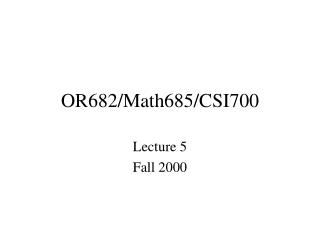OR682/Math685/CSI700

# OR682/Math685/CSI700 - PowerPoint PPT Presentation

OR682/Math685/CSI700. Lecture 5 Fall 2000. Nonlinear Equations. Solving f ( x ) = 0 (1 equation, n equations) Assume that [# of equations] = [# of variables] Closely related to: minimize F ( x ) Solve:  F ( x ) = 0I am the owner, or an agent authorized to act on behalf of the owner, of the copyrighted work described.
Download Presentation## OR682/Math685/CSI700

An Image/Link below is provided (as is) to download presentation

Download Policy: Content on the Website is provided to you AS IS for your information and personal use and may not be sold / licensed / shared on other websites without getting consent from its author.While downloading, if for some reason you are not able to download a presentation, the publisher may have deleted the file from their server.

- - - - - - - - - - - - - - - - - - - - - - - - - - E N D - - - - - - - - - - - - - - - - - - - - - - - - - -
Presentation Transcript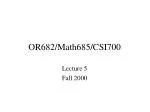### OR682/Math685/CSI700

Lecture 5

Fall 2000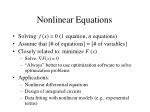Nonlinear Equations
• Solving f (x) = 0 (1 equation, n equations)
• Assume that [# of equations] = [# of variables]
• Closely related to: minimize F (x)
• Solve: F(x) = 0
• “Always” better to use optimization software to solve optimization problems
• Applications:
• Nonlinear differential equations
• Design of integrated circuits
• Data fitting with nonlinear models (e.g., exponential terms)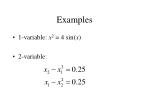Examples
• 1-variable: x2 = 4 sin(x)
• 2-variable: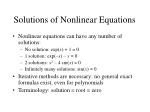Solutions of Nonlinear Equations
• Nonlinear equations can have any number of solutions:
• No solution: exp(x) + 1 = 0
• 1 solution: exp(–x) – x = 0
• 2 solutions: x2 – 4 sin(x) = 0
• Infinitely many solutions: sin(x) = 0
• Iterative methods are necessary: no general exact formulas exist, even for polynomials
• Terminology: solution = root = zero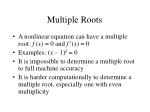Multiple Roots
• A nonlinear equation can have a multiple root: f (x) = 0 and f(x) = 0
• Examples: (x – 1)k = 0
• It is impossible to determine a multiple root to full machine accuracy
• It is harder computationally to determine a multiple root, especially one with even multiplicity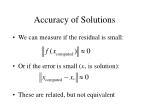Accuracy of Solutions
• We can measure if the residual is small:
• Or if the error is small (x* is solution):
• These are related, but not equivalent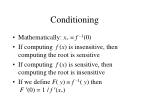Conditioning
• Mathematically: x* = f –1(0)
• If computing f (x) is insensitive, then computing the root is sensitive
• If computing f (x) is sensitive, then computing the root is insensitive
• If we define F( y) f –1( y) thenF (0) = 1 / f (x*)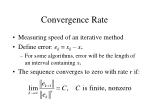Convergence Rate
• Measuring speed of an iterative method
• Define error: ek = xk– x*
• For some algorithms, error will be the length of an interval containing x*
• The sequence converges to zero with rate r if: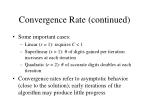Convergence Rate (continued)
• Some important cases:
• Linear (r = 1): requires C < 1
• Superlinear (r > 1): # of digits gained per iteration increases at each iteration
• Quadratic (r = 2): # of accurate digits doubles at each iteration
• Convergence rates refer to asymptotic behavior (close to the solution); early iterations of the algorithm may produce little progress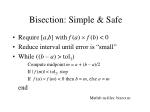Bisection: Simple & Safe
• Require [a,b] with f (a)  f (b) < 0
• Reduce interval until error is “small”
• While ((b – a) > tol1)

Compute midpoint m = a + (b– a)/2

If | f (m)| < tol2, stop

If f (a) f (m) < 0 then b = m, else a = m

end

Matlab m-files: bisect.mBisection, Continued
• Interval reduced by ½ each iteration
• Linear convergence (r = 1, C = ½)
• Bisection approximates f (x) by the line through [a,sign( f (a))] and [b,sign( f (b))] and determines the point m where this line is zero
• This is a crude model of f (x)
• What about multiple roots?

Matlab m-files: bisect_model.mNewton’s Method
• Approximate f (x) by its Taylor series:
• Find point where line is zero:
• Repeat this computation to get Newton’s method:

Matlab m-files: newton_model.m, newton.mNewton’s Method: Convergence
• Note: ek = xk– x* so x* = xk – ek. Thus
• Quadratic convergence (r = 2) if f(x*)  0Secant Method
• Goal: reduce iteration cost of Newton’s method
• Approximate f(x) by finite difference:
• Superlinear convergence (r 1.6)Safeguarded Methods
• Newton, secant methods:
• Fast close to solution
• Potentially unreliable (esp. away from solution)
• Bisection (and other) methods:
• Slow to converge
• Reliable
• Safeguarded method:
• Monitor performance of fast method
• Use slow, safe method to guarantee convergence
• Near solution, the slow method usually not neededSystems of Nonlinear Equations
• Much more difficult than scalar case
• Theoretical analysis harder, behavior of roots potentially stranger
• No absolutely safe, reliable method
• Costs rise rapidly with # of variables
• Can only guarantee that algorithm converges to a solution of:Newton’s Method
• In n dimensions:

where (J = Jacobian matrix)

• Quadratic convergence rate (if assumptions satisfied)

Matlab m_files: newton_s.mNewton’s Method (continued)
• Computational costs
• O(n2) to compute Jacobian
• O(n3) to solve Newton equations
• Alternative methods
• Analogs of secant method
• Safeguards
• Essential to guarantee convergence
• “line search” or “trust region”Matlab Software
• 1-variable: fzero
• n-variable: fsolveFor Next Class
• Homework: see web site